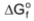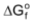# Free Energy Change And Spontaneity Of A Reaction

## Free Energy Change And Spontaneity Of A Reaction

Every reaction proceeds with a decrease in free energy. The free energy change in a process is expressed by ΔG.

IfΔG = −ve, reaction goes in the forward direction

ΔG = +ve, reaction goes in the backward direction

ΔG = 0 ,reaction is at equilibrium

Free energy, G  denotes the self intrinsic electrostatic potential energy of a system. This means that in any molecule if we calculate the total electrostatic potential energy of all the charges due to all the other charges, we get what is called the free energy of the molecule. It tells us about the stability of a molecule with respect to another molecule. Lesser the free energy of a molecule more stable it is.

ΔG is the free energy change at any given concentration of reactants and products. If all the reactants and products are taken at a concentration of one mol per litre, the free energy change of the reaction is called ΔG° (standard free energy change). One must understand ΔG° is not the free energy change at equilibrium.

ΔG° = Σof products − Σof reactants

andΔG =Σ ΔGf of products − Σ ΔGf of reactants

Relation between ΔG° and K

ΔG° = −RT ln K

T is always in Kelvin, and if R is in joules, ΔG° will be in joules, and if R is calories then ΔG° will be in calories.

[Note: K may either be KC or KP or any other equilibrium constant. The use of this relation between ΔG° and K will be extensively seen in the topic, electrochemistry.]